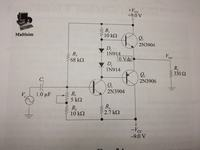# Class AB amp, how does thing even work with a negative base voltage?

Status
Not open for further replies.

#### maark6000

##### Member level 5
Not sure I understand how this circuit operates. The voltage divider is creating a negative voltage at the base of Q3 (-6.75 v), which from what I've been taught would not forward bias the transistor (+0.7 v ?), and thus there would be no current in the middle leg.

The circuit does work, I was able to use the pot to balance the node between Q1 and Q2, and the circuit put out a 8.5 v (pp) signal before clipping.

what am I missing?

thanks as always!Not sure I understand how this circuit operates. The voltage divider is creating a negative voltage at the base of Q3 (-6.75 v), which from what I've been taught would not forward bias the transistor (+0.7 v ?),

Transistor operation requires a voltage BETWEEN base and emitter of approx. 0.7 Volts. Look at the emitter potential!

ah! there it is. thank you. with the small 2.7k emitter resistor, the emitter voltage would be around -7.5 v, enough to forward bias the transistor. So in calculating IE (VE/RE), I would get -2.78 mA. But what does the negative sign mean... current is still flowing from Q3 down to -Vcc, right? and if IE roughly equals IC, is IC also -2.78 mA?

FYI the centre of the two diodes is always very close to 0V, else one output transistor is turned on more then the other, with the output terminal then not being at 0V. So the collector of Q3 is .7(diode volt drop) more negative then 0V i.e.- .7V.
Frank

Status
Not open for further replies.